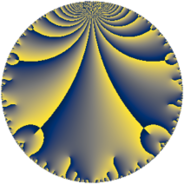Properties

 Label 245.6.aLevel $245$ Weight $6$ Character orbit 245.a Rep. character $\chi_{245}(1,\cdot)$ Character field $\Q$ Dimension $69$ Newform subspaces $13$ Sturm bound $168$ Trace bound $3$

Related objects

Defining parameters

 Level: $$N$$ $$=$$ $$245 = 5 \cdot 7^{2}$$ Weight: $$k$$ $$=$$ $$6$$ Character orbit: $$[\chi]$$ $$=$$ 245.a (trivial) Character field: $$\Q$$ Newform subspaces: $$13$$ Sturm bound: $$168$$ Trace bound: $$3$$ Distinguishing $$T_p$$: $$2$$, $$3$$

Dimensions

The following table gives the dimensions of various subspaces of $$M_{6}(\Gamma_0(245))$$.

Total New Old
Modular forms 148 69 79
Cusp forms 132 69 63
Eisenstein series 16 0 16

The following table gives the dimensions of the cuspidal new subspaces with specified eigenvalues for the Atkin-Lehner operators and the Fricke involution.

$$5$$$$7$$FrickeDim.
$$+$$$$+$$$$+$$$$16$$
$$+$$$$-$$$$-$$$$19$$
$$-$$$$+$$$$-$$$$18$$
$$-$$$$-$$$$+$$$$16$$
Plus space$$+$$$$32$$
Minus space$$-$$$$37$$

Trace form

 $$69 q + 6 q^{2} - 40 q^{3} + 1168 q^{4} - 25 q^{5} + 72 q^{6} - 348 q^{8} + 5765 q^{9} + O(q^{10})$$ $$69 q + 6 q^{2} - 40 q^{3} + 1168 q^{4} - 25 q^{5} + 72 q^{6} - 348 q^{8} + 5765 q^{9} - 150 q^{10} + 1420 q^{11} - 3796 q^{12} - 690 q^{13} + 1000 q^{15} + 19124 q^{16} - 1822 q^{17} + 7202 q^{18} - 1916 q^{19} + 700 q^{20} - 1560 q^{22} + 1436 q^{23} + 15124 q^{24} + 43125 q^{25} + 8808 q^{26} - 1732 q^{27} + 994 q^{29} - 7800 q^{30} - 1692 q^{31} + 500 q^{32} + 26628 q^{33} + 23364 q^{34} + 94824 q^{36} + 30054 q^{37} - 700 q^{38} - 50040 q^{39} - 12600 q^{40} - 14246 q^{41} - 31096 q^{43} - 15132 q^{44} - 125 q^{45} + 17468 q^{46} - 35116 q^{47} - 13332 q^{48} + 3750 q^{50} + 10056 q^{51} - 11776 q^{52} - 8634 q^{53} + 21628 q^{54} - 24100 q^{55} - 108112 q^{57} - 45776 q^{58} - 67192 q^{59} + 80900 q^{60} - 7230 q^{61} + 97320 q^{62} + 238988 q^{64} + 26550 q^{65} + 108732 q^{66} - 66040 q^{67} - 37900 q^{68} - 129780 q^{69} + 40200 q^{71} + 95728 q^{72} + 88014 q^{73} - 114536 q^{74} - 25000 q^{75} + 101676 q^{76} + 146472 q^{78} - 118008 q^{79} + 48400 q^{80} + 280549 q^{81} - 360968 q^{82} + 105540 q^{83} - 61550 q^{85} + 227696 q^{86} + 241348 q^{87} - 47344 q^{88} + 306526 q^{89} - 163450 q^{90} + 65020 q^{92} + 155112 q^{93} - 309980 q^{94} + 82100 q^{95} + 256188 q^{96} - 72870 q^{97} + 1230636 q^{99} + O(q^{100})$$

Decomposition of $$S_{6}^{\mathrm{new}}(\Gamma_0(245))$$ into newform subspaces

Label Dim. $$A$$ Field CM Traces A-L signs $q$-expansion
$a_{2}$ $a_{3}$ $a_{5}$ $a_{7}$ 5 7
245.6.a.a $1$ $39.294$ $$\Q$$ None $$-8$$ $$-1$$ $$-25$$ $$0$$ $+$ $-$ $$q-8q^{2}-q^{3}+2^{5}q^{4}-5^{2}q^{5}+8q^{6}+\cdots$$
245.6.a.b $1$ $39.294$ $$\Q$$ None $$2$$ $$4$$ $$-25$$ $$0$$ $+$ $-$ $$q+2q^{2}+4q^{3}-28q^{4}-5^{2}q^{5}+8q^{6}+\cdots$$
245.6.a.c $2$ $39.294$ $$\Q(\sqrt{65})$$ None $$1$$ $$-3$$ $$50$$ $$0$$ $-$ $-$ $$q+\beta q^{2}+(-3+3\beta )q^{3}+(-2^{4}+\beta )q^{4}+\cdots$$
245.6.a.d $3$ $39.294$ 3.3.577880.1 None $$-6$$ $$-26$$ $$75$$ $$0$$ $-$ $-$ $$q+(-2+\beta _{1})q^{2}+(-9-\beta _{2})q^{3}+(38+\cdots)q^{4}+\cdots$$
245.6.a.e $4$ $39.294$ $$\mathbb{Q}[x]/(x^{4} - \cdots)$$ None $$7$$ $$-14$$ $$-100$$ $$0$$ $+$ $-$ $$q+(2-\beta _{1})q^{2}+(-4+\beta _{1}-\beta _{3})q^{3}+\cdots$$
245.6.a.f $5$ $39.294$ $$\mathbb{Q}[x]/(x^{5} - \cdots)$$ None $$-3$$ $$-7$$ $$125$$ $$0$$ $-$ $-$ $$q+(-1+\beta _{1})q^{2}+(-1-\beta _{1}-\beta _{2})q^{3}+\cdots$$
245.6.a.g $5$ $39.294$ $$\mathbb{Q}[x]/(x^{5} - \cdots)$$ None $$-3$$ $$7$$ $$-125$$ $$0$$ $+$ $-$ $$q+(-1+\beta _{1})q^{2}+(1+\beta _{1}+\beta _{2})q^{3}+\cdots$$
245.6.a.h $6$ $39.294$ $$\mathbb{Q}[x]/(x^{6} - \cdots)$$ None $$-5$$ $$-20$$ $$150$$ $$0$$ $-$ $-$ $$q+(-1+\beta _{1})q^{2}+(-3-\beta _{1}+\beta _{3})q^{3}+\cdots$$
245.6.a.i $6$ $39.294$ $$\mathbb{Q}[x]/(x^{6} - \cdots)$$ None $$-5$$ $$20$$ $$-150$$ $$0$$ $+$ $+$ $$q+(-1+\beta _{1})q^{2}+(3+\beta _{1}-\beta _{3})q^{3}+\cdots$$
245.6.a.j $8$ $39.294$ $$\mathbb{Q}[x]/(x^{8} - \cdots)$$ None $$3$$ $$-2$$ $$200$$ $$0$$ $-$ $+$ $$q+\beta _{1}q^{2}-\beta _{3}q^{3}+(5^{2}+\beta _{1}+\beta _{2})q^{4}+\cdots$$
245.6.a.k $8$ $39.294$ $$\mathbb{Q}[x]/(x^{8} - \cdots)$$ None $$3$$ $$2$$ $$-200$$ $$0$$ $+$ $-$ $$q+\beta _{1}q^{2}+\beta _{3}q^{3}+(5^{2}+\beta _{1}+\beta _{2})q^{4}+\cdots$$
245.6.a.l $10$ $39.294$ $$\mathbb{Q}[x]/(x^{10} - \cdots)$$ None $$10$$ $$-58$$ $$-250$$ $$0$$ $+$ $+$ $$q+(1-\beta _{1})q^{2}+(-6+\beta _{3})q^{3}+(18-\beta _{1}+\cdots)q^{4}+\cdots$$
245.6.a.m $10$ $39.294$ $$\mathbb{Q}[x]/(x^{10} - \cdots)$$ None $$10$$ $$58$$ $$250$$ $$0$$ $-$ $+$ $$q+(1-\beta _{1})q^{2}+(6-\beta _{3})q^{3}+(18-\beta _{1}+\cdots)q^{4}+\cdots$$

Decomposition of $$S_{6}^{\mathrm{old}}(\Gamma_0(245))$$ into lower level spaces

$$S_{6}^{\mathrm{old}}(\Gamma_0(245)) \cong$$ $$S_{6}^{\mathrm{new}}(\Gamma_0(5))$$$$^{\oplus 3}$$$$\oplus$$$$S_{6}^{\mathrm{new}}(\Gamma_0(7))$$$$^{\oplus 4}$$$$\oplus$$$$S_{6}^{\mathrm{new}}(\Gamma_0(35))$$$$^{\oplus 2}$$$$\oplus$$$$S_{6}^{\mathrm{new}}(\Gamma_0(49))$$$$^{\oplus 2}$$